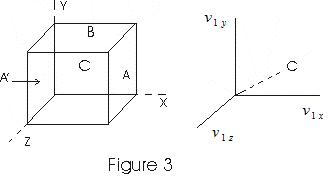# Pressure of Gas

## Pressure of gas

• Consider a cubical vessel with perfectly elastic walls containing large number of molecules say N let l be the dimension of each side of the cubical vessel.
• v1x , v1y, v1z be the x, y, and z component of a molecule with velocity v.
• Consider the motion of molecule in the direction perpendicular to the face of cubical vessel.
• Molecule strikes the face A with a velocity v1x and rebounds with the same velocity in the backward direction as the collisions are perfectly elastic.• If m is the mass of molecule, the change in Momentum during collision is
mv1x - (-mv1x) = 2 mv1x          (1)
• The distance travelled parallel to x-axis an is between A to A´ and when molecule rebounds from A´ and travel towards A is 2L
• Time taken by molecule to go to face A´ and then comeback to A is
Δt = 2l/v1x
• Number of impacts of this molecule with A in unit time is
n = I/Δt = v1x/ 2l               (2)
Rate of change of momentum is
ΔF = ΔP/Δt
=nΔP
from (1) and (2)
ΔF = mv1x2 / l
this is the force exerted on wall A due to this molecule.
• Force on wall A due to all other molecules
F = Σmv1x2/L           (3)
• As all directions are equivalent
Σv1x2=Σv1y2=Σv1z2
Σv1x2= 1/3Σ((v1x)2 + (v1y)2 +( v1z)2 )
= 1/3 Σv12
Thus     F = (m/3L) Σv12
• N is total no. of molecules in the container so
F = (mN/3L) (Σ(v1)2/N)
• Pressure is force per unit area so
P = F/L2
=(M/3L3)(Σ(v1)2/N)

where ,M is the total mass of the gas and if ρ is the density of gas then
P=ρΣ(v1)2/3N
since Σ(v1)2/N is the average of squared speeds and is written as vmq2 known as mean square speed
Thus, vrms=√(Σ(v1)2/N) is known as root mean squared speed rms-speed and vmq2 = (vrms)2

• Pressure thus becomes
P = (1/3)ρvmq2                     (4)
or     PV = (1/3) Nmvmq2                     (5)
from equation (4) rms speed is given as
vrms = √(3P/ρ)
= √(3PV/M)
(6)